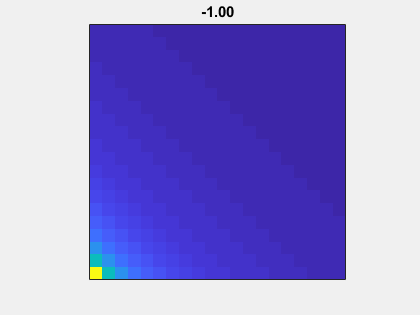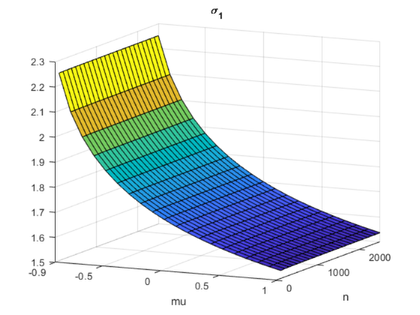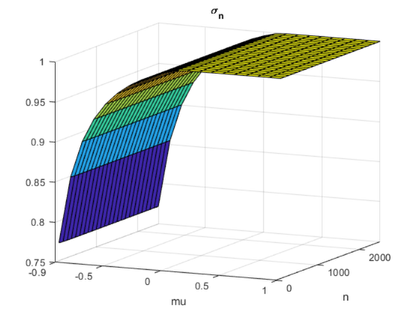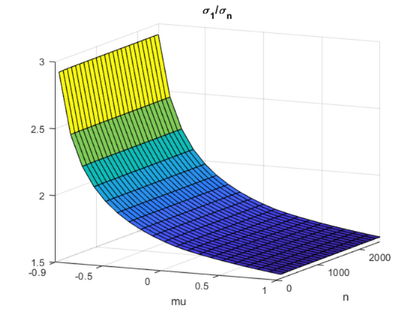# A Matrix for the New HPL-AI Benchmark

My colleagues are looking for a matrix to be used in a new benchmark. They've come to the right place.

### Contents

#### Email

A couple of weeks ago I got this email from Jack Dongarra at the University of Tennessee.

For this new HPL-AI benchmark, I'm looking for a matrix that is not symmetric, is easily generated (roughly O(n^2) ops to generate), is dense, doesn’t require pivoting to control growth, and has a smallish condition number (<O(10^5)).

There are two steps for the benchmark:

1) do LU on the matrix in short precision and compute an approximation solution x. (On Nvidia this is 6 times faster in half precision then in DP.)

2) do GMRES on with L and U as preconditions with x as the starting point (this usually takes 3-4 iterations to converge.)

The benchmark details are here: http://bit.ly/hpl-ai.

Any ideas for a matrix?

#### Symmetric Hilbert Matrix

One of the first matrices I ever studied was the Hilbert matrix. With the singleton expansion features now in MATLAB, the traditional symmetric Hilbert matrix can be generated by

  format rat
n = 5;
i = 1:n
j = i'
H = 1./(i+j-1)

i =
1              2              3              4              5
j =
1
2
3
4
5
H =
1              1/2            1/3            1/4            1/5
1/2            1/3            1/4            1/5            1/6
1/3            1/4            1/5            1/6            1/7
1/4            1/5            1/6            1/7            1/8
1/5            1/6            1/7            1/8            1/9


When you add the row vector i to the column vector j, the resulting i+j is a matrix. Compare this with the inner product, i*j, which produces a scalar, and the outer product, j*i, which produces another matrix. The expression i+j can be thought of as an "outer sum".

Two more scalar expansions complete the computation; i+j-1 subtracts 1 from each element of the sum and 1./(i+j-1) divides 1 by each element in the denominator. Both of these scalar expansions have been in MATLAB from its earliest days.

The elements of Hilbert matrices depend only on the sum i+j, so they are constant on the antidiagonals. Such matrices are known as Cauchy matrices. There are fast algorithms for solving system of equations involving Cauchy matrices in $O(n^2)$ time.

#### Nonsymmetric Hilbert Matrix

Some years ago, I wanted a nonsymmetric test matrix, so I changed the plus sign in the denominator of the Hilbert matrix to a minus sign. But this created a division by zero along the subdiagonal, so I also changed the -1 to +1/2. I call this the "nonsymmetric Hilbert matrix."

   H = 1./(i-j+1/2)

H =
2              2/3            2/5            2/7            2/9
-2              2              2/3            2/5            2/7
-2/3           -2              2              2/3            2/5
-2/5           -2/3           -2              2              2/3
-2/7           -2/5           -2/3           -2              2


The elements depend only on i-j and so are constant on the diagonals. The matrices are Toeplitz and, again, such systems can be solved with $O(n^2)$ operations.

I was astonished when I first computed the singular values of nonsymmetric Hilbert matrices -- most of them were nearly equal to $\pi$. Already with only the 5-by-5 we see five decimal places.

   format long
sigma = svd(H)

sigma =
3.141589238341321
3.141272220456508
3.129520593545258
2.921860834913688
1.461864493324889


This was a big surprise. The eventual explanation, provided by Seymour Parter, involves a theorem of Szego from the 1920's relating the eigenvalues of Toeplitz matrices to Fourier series and square waves.

#### Benchmark Matrix

I have been working with Jack's colleague Piotr Luszczek. This is our suggestion for the HPL-AI Benchmark Matrix. It depends upon two parameters, n and mu. As mu goes from 1 to -1, the core of this matrix goes from the symmetric to the nonsymmetric Hilbert matrix. Adding n in the denominator and 1's on the diagonal produces diagonal dominance.

   A = 1./(i + mu*j + n) + double(i==j);

   clear A
type A

function Aout = A(n,mu)
% A(n,mu).  Matrix for the HPL-AI Benchmark.  mu usually in [-1, 1].
i = 1:n;
j = i';
Aout = 1./(i+mu*j+n) + eye(n,n);
end



If mu is not equal to +1, this matrix is not Cauchy. And, if mu is not equal to -1, it is not Toeplitz. Furthermore, if mu is greater than -1, it is diagonally dominant and, as we shall see, very well-conditioned.

#### Example

Here is the 5x5 with rational output. When mu is +1, we get a section of a symmetric Hilbert matrix plus 1's on the diagonal.

   format rat
mu = 1;
A(n,mu)

ans =
8/7            1/8            1/9            1/10           1/11
1/8           10/9            1/10           1/11           1/12
1/9            1/10          12/11           1/12           1/13
1/10           1/11           1/12          14/13           1/14
1/11           1/12           1/13           1/14          16/15


And when mu is -1, the matrix is far from symmetric. The element in the far southwest corner almost dominates the diagonal.

   mu = -1;
A(n,mu)

ans =
6/5            1/6            1/7            1/8            1/9
1/4            6/5            1/6            1/7            1/8
1/3            1/4            6/5            1/6            1/7
1/2            1/3            1/4            6/5            1/6
1              1/2            1/3            1/4            6/5


#### Animation

Here is an animation of the matrix without the addition of eye(n,n). As mu goes to -1 to 1, A(n,mu)-eye(n,n) goes from nonsymmetric to symmetric.#### Gaussian elimination

If mu > -1, the largest element is on the diagonal and lu(A(n,mu)) does no pivoting.

   format short
mu = -1
[L,U] = lu(A(n,mu))

mu = 1
[L,U] = lu(A(n,mu))

mu =
-1
L =
1.0000         0         0         0         0
0.2083    1.0000         0         0         0
0.2778    0.1748    1.0000         0         0
0.4167    0.2265    0.1403    1.0000         0
0.8333    0.3099    0.1512    0.0839    1.0000
U =
1.2000    0.1667    0.1429    0.1250    0.1111
0    1.1653    0.1369    0.1168    0.1019
0         0    1.1364    0.1115    0.0942
0         0         0    1.1058    0.0841
0         0         0         0    1.0545
mu =
1
L =
1.0000         0         0         0         0
0.1094    1.0000         0         0         0
0.0972    0.0800    1.0000         0         0
0.0875    0.0729    0.0626    1.0000         0
0.0795    0.0669    0.0580    0.0513    1.0000
U =
1.1429    0.1250    0.1111    0.1000    0.0909
0    1.0974    0.0878    0.0800    0.0734
0         0    1.0731    0.0672    0.0622
0         0         0    1.0581    0.0542
0         0         0         0    1.0481


#### Condition

Let's see how the 2-condition number, $\sigma_1/\sigma_n$, varies as the parameters vary. Computing the singular values for all the matrices in the following plots takes a little over 10 minutes on my laptop.

#### sigma_1

   load HPLAI.mat s1 sn n mu

surf(mu,n,s1)
view(25,12)
xlabel('mu')
ylabel('n')
set(gca,'xlim',[-0.9 1.0], ...
'xtick',[-0.9 -0.5 0 0.5 1.0], ...
'ylim',[0 2500])
title('\sigma_1')The colors vary as mu varies from -0.9 up to 1.0. I am staying away from mu = -1.0 where the matrix looses diagonal dominance. The other horizontal axis is the matrix order n. I have limited n to 2500 in these experiments.

The point to be made is that if mu > -0.9, then the largest singular value is bounded, in fact sigma_1 < 2.3.

#### sigma_n

   surf(mu,n,sn)
view(25,12)
xlabel('mu')
ylabel('n')
set(gca,'xlim',[-0.9 1.0], ...
'xtick',[-0.9 -0.5 0 0.5 1.0], ...
'ylim',[0 2500])
title('\sigma_n')If mu > -0.9, then the smallest singular value is bounded away from zero, in fact sigma_n > .75.

#### sigma_1/sigma_n

   surf(mu,n,s1./sn)
view(25,12)
xlabel('mu')
ylabel('n')
set(gca,'xlim',[-0.9 1.0], ...
'xtick',[-0.9 -0.5 0 0.5 1.0], ...
'ylim',[0 2500])
title('\sigma_1/\sigma_n')If mu > -0.9, then the condition number in the 2-norm is bounded, sigma_1/sigma_n < 2.3/.75 $\approx$ 3.0 .

#### Overflow

The HPL-AI benchmark would begin by computing the LU decomposition of this matrix using 16-bit half-precision floating point arithmetic, FP16. So, there is a signigant problem if the matrix order n is larger than 65504. This value is realmax, the largest number that can be represented in FP16. Any larger value of n overflows to Inf.

Some kind of scaling is going to have to be done for n > 65504. Right now, I don't see what this might be.

The alternative half-precision format to FP16 is Bfloat16, which has three more bits in the exponent to give realmax = 3.39e38. There should be no overflow problems while generating this matrix with Bfloat16.

#### Thanks

Thanks to Piotr Luszczek for working with me on this. We have more work to do.

Published with MATLAB® R2019b

|